# ylim

Set or query y-axis limits

## Syntax

``ylim(limits)``
``ylim(limitmethod)``
``ylim(limitmode)``
``yl = ylim``
``limmethod = ylim("method")``
``limmode = ylim("mode")``
``___ = ylim(target,___)``

## Description

### Specify Limits

example

````ylim(limits)` sets the y-axis limits for the current axes or chart. Specify `limits` as a two-element vector of the form `[ymin ymax]`, where `ymax` is greater than `ymin`.```

example

````ylim(limitmethod)` specifies the limit method MATLAB® uses for automatic limit selection. Specify the limit method as `"tickaligned"`, `"tight"`, or `"padded"`. MATLAB sets the `YLimitMethod` property of the axes to the value you specify. The limit method is not supported for standalone visualizations.You can specify the `limitmethod` argument without parentheses. For example, `ylim tight` enables tight y-axis limits.```

example

````ylim(limitmode)` specifies automatic or manual limit selection. The `limitmode` can have either of two values: `"auto"` — Enable automatic limit selection. MATLAB selects the limits based on the range of your data and the value of the `YLimitMethod` property of the axes. If you plot into the axes multiple times, the limits update to encompass all the data.`"manual"` — Freeze the y-axis limits at their current value. You can specify the `limitmode` argument without parentheses. For example, `ylim auto` enables automatic limit selection.```

### Query Limits

example

````yl = ylim` returns the current limits as a two-element vector.```
````limmethod = ylim("method")` returns the current y-axis limits method, which can be `'tickaligned'`, `'tight'`, or `'padded'`.```
````limmode = ylim("mode")` returns the current y-axis limits mode, which is either `'auto'` or `'manual'`. By default, the mode is automatic unless you specify limits or set the mode to manual.```

### Specify Target Axes or Chart

example

````___ = ylim(target,___)` uses the axes or standalone visualization specified by `target` instead of the current axes. Specify `target` as the first input argument for any of the previous syntaxes. You can include an output argument if the original syntax supports an output argument. Use quotes around the mode inputs, for example, `ylim(target,"auto")`.```

## Examples

collapse all

Plot a line and set the y-axis limits to range from -2 to 2.

```x = linspace(0,10); y = sin(x); plot(x,y) ylim([-2 2])```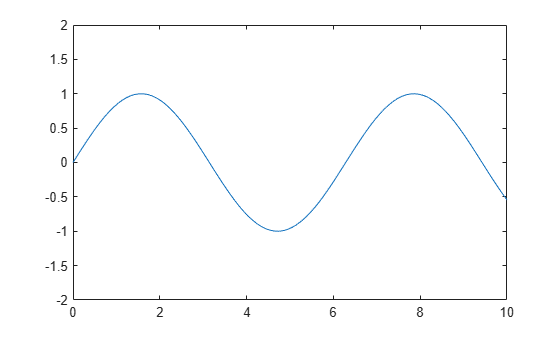Create a surface plot and show only y values greater than 0. Specify the minimum y-axis limit as 0 and let MATLAB choose the maximum limit.

```[X,Y,Z] = peaks; surf(X,Y,Z) ylim([0 inf])```Create a horizontal bar chart with dates along the y-axis. Set the y-axis limits to range from June 1, 2014 to June 10, 2014.

```t = datetime(2014,06,1) + caldays(0:20); y = rand(21,1); barh(t,y) tstart = datetime(2014,06,1); tend = datetime(2014,06,10); ylim([tstart tend])```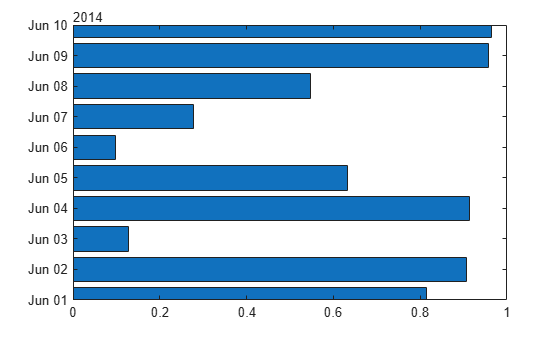Create a line plot.

```x = 1:5; y = [-2.7 2 -1 4.2 0]; plot(x,y)```Change the limit method to `'tight'`.

`ylim tight`Starting in R2019b, you can display a tiling of plots using the `tiledlayout` and `nexttile` functions. Call the `tiledlayout` function to create a 2-by-1 tiled chart layout. Call the `nexttile` function to create the axes objects `ax1` and `ax2`. Plot data into each axes. Then set the y-axis limits for the bottom plot by specifying `ax2` as the first input argument to `ylim`.

```tiledlayout(2,1) x = linspace(0,10,1000); y = sin(10*x).*exp(.5*x); ax1 = nexttile; plot(ax1,x,y) ax2 = nexttile; plot(ax2,x,y) ylim(ax2,[-10 10])```Use manual mode to maintain the current y-axis limits when you add more plots to the axes.

First, plot a line.

```x = linspace(0,10); y = sin(x); plot(x,y)```Set the y-axis limits mode to manual so that the limits to not change. Use `hold on` to add a second plot to the axes.

```ylim manual hold on y2 = 2*sin(x); plot(x,y2) hold off```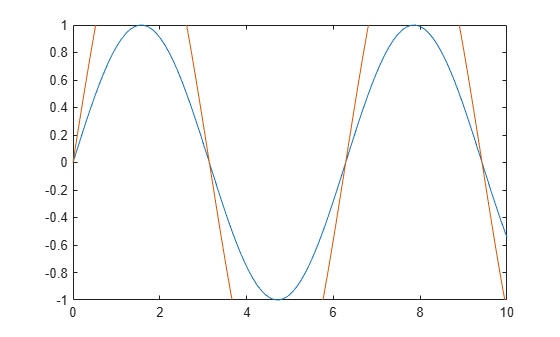The y-axis limits do not update to incorporate the new plot.

Switch back to automatically updated limits by resetting the mode to automatic.

`ylim auto`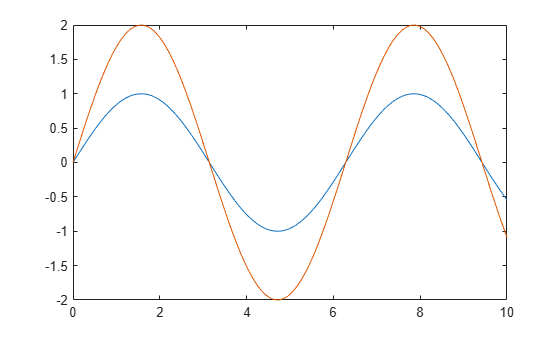Create a scatter plot of random data. Return the values of the y-axis limits.

```x = randn(50,1); y = randn(50,1); scatter(x,y)````yl = ylim`
```yl = 1×2 -2 3 ```

## Input Arguments

collapse all

Minimum and maximum limits, specified as a two-element vector of the form `[ymin ymax]`, where `ymax` is greater than `ymin`. You can specify the limits as numeric, categorical, datetime, or duration values. However, the type of values that you specify must match the type of values along the y-axis.

You can specify both limits, or specify one limit and let MATLAB automatically calculate the other. For an automatically calculated minimum or maximum limit, use `-inf` or `inf`, respectively. MATLAB uses the `'tight'` limit method to calculate the corresponding limit.

Example: `ylim([0 1])`

Example: `ylim([-inf 1])`

Example: `ylim([0 inf])`

Data Types: `single` | `double` | `int8` | `int16` | `int32` | `int64` | `uint8` | `uint16` | `uint32` | `uint64` | `categorical` | `datetime` | `duration`

Limit selection method, specified as a value from the table.

The examples in the table show the approximate appearance for each method. Your results might differ depending on your data, the size of the axes, and the type of plot you create.

ValueDescriptionExample
`"tickaligned"`

In general, align the edges of the axes box with the tick marks that are closest to your data without excluding any data. The appearance might vary depending on the type of data you plot and the type of chart you create.`"tight"`

Fit the axes box tightly around the data by setting the axis limits to the data range.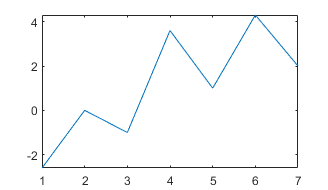`"padded"`

Fit the axes box around the data with a thin margin of padding on each side. The width of the margin is approximately 7% of the data range.Note

• The limit method has no effect when the `YLimMode` property of the axes is set to `"manual"`.

• Specifying the limit method is not supported for standalone visualizations such as `heatmap`.

Limit mode, specified as one of the following values:

• `"auto"` — Enable automatic limit selection, which is based on the total span of the data and the value of the `YLimitMethod` property of the axes. If you plot into the axes multiple times, the limits update to encompass all the data. You can use this option if you change the limits and want to set them back to the default values.

• `"manual"` — Freeze the limits at the current values. Use this option if you want to retain the current limits when adding new data to the axes using the ```hold on``` command.

When you specify this argument, MATLAB sets the `YLimMode` property of the axes to the value you specify. However, the `YLimMode` property changes to `"manual"` whenever you set the y-axis limits explicitly, either by calling `ylim(limits)`, or by setting the value of the `YLim` property on the axes.

Target axes or chart, specified as one of the following:

• An `Axes` object.

• A standalone visualization that has a `YLimits` property, such as a `heatmap` chart.

• An array of axes or standalone visualizations that belong to the same class. To determine the class, use the `class` function.

If you do not specify this argument, then `ylim` sets the limits on the graphics object returned by the `gca` command.

## Output Arguments

collapse all

Current limits, returned as a two-element vector of the form ```[ymin ymax]```.

Querying the limits returns the `YLim` or `YLimits` property value for the corresponding `Axes` or graphics object.

Current limits method, returned as one of these values:

• `'tickaligned'` — In general, align the edges of the axes box with the tick marks that are closest to your data without excluding any data. The appearance might vary depending on the type of data you plot and the type of chart you create.

• `'tight'` — Fit the axes box tightly around the data by setting the axis limits to the data range.

• `'padded'` — Fit the axes box around the data with a thin margin of padding on each side. The width of the margin is approximately 7% of the data range.

Querying the y-axis limits method returns the `YLimitMethod` property value for the corresponding `Axes` object.

Current limits mode, returned as one of these values:

• `'auto'` — Automatically determine the limits.

• `'manual'` — Use manually specified limits that do not update to reflect changes in the data.

Querying the y-axis limits mode returns the `YLimMode` property value for the corresponding `Axes` object.

## Algorithms

The `ylim` function sets and queries several axes properties related to the y-axis limits.

• `YLim` — Property that stores the y-axis limits.

• `YLimMode` — Property that stores the y-axis limits mode. When you set the y-axis limits, this property changes to `"manual"`.

• `YLimitMethod` — Property that controls how the y-axis limits are calculated when the `YLimMode` property is set to `"auto"`.

## Version History

Introduced before R2006a

expand all